# PROPER Function – Convert Text – Excel, VBA, & Google Sheets

This tutorial demonstrates how to use the Excel PROPER Function in Excel to convert text to proper case.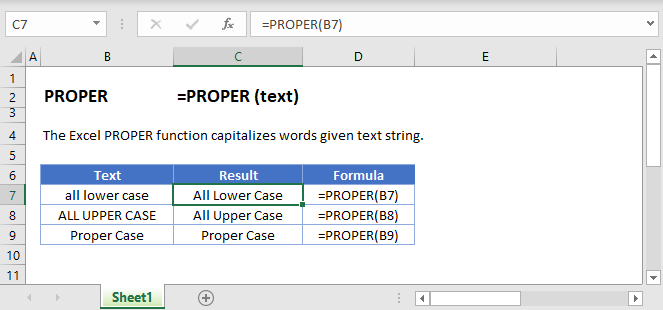## PROPER Function Overview

The PROPER Function Converts entire text string to proper case. The first letter in each word is capitalized.

To use the PROPER Excel Worksheet Function, select a cell and type: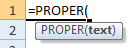(Notice how the formula inputs appear)

### PROPER function Syntax and inputs:

`=PROPER(text)`

text – A string of text.

## What Is the PROPER Function?

The Excel PROPER Function takes a given string as an input, and then returns that string with the first letter of each word in upper case, but every other letter in lower case.

## How to Use the PROPER Function

You can use the Excel PROPER function like this:

`=PROPER(“bruce springsteen”)`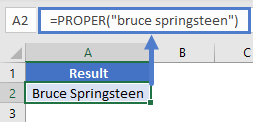This would return the following as above screen shot.

Bruce Springsteen

PROPER is useful when you have a column of data with inconsistent formatting.

For example, imagine you have a spreadsheet of musical artists, but the names haven’t been entered correctly. Some are upper case, some are lower, others are a mix of upper and lower… it’s a mess. You can use PROPER to fix this, as shown here:

`=PROPER(A2)`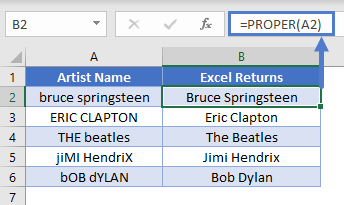### PROPER Will Affect Every Word

Note that PROPER will affect every word in the text string. Depending on the data you have, this may or may not be desirable, for example: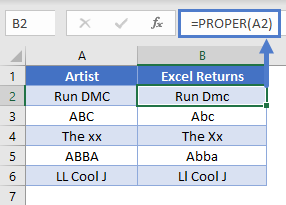### How PROPER Handles Punctuation and Numbers

The Excel PROPER Function does not change numbers, punctuation, or special characters. However, it does treat them as spaces. That means PROPER will capitalize any letter that directly follows a number or a punctuation mark.

Check out these examples below: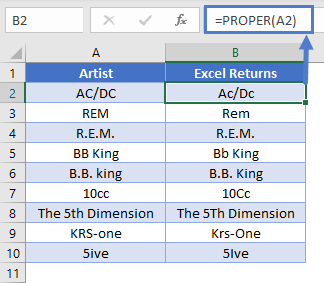### Keeping State Codes Capitalized

If you only want to use PROPER on a portion of the text, and keep the rest in either upper or lower case, this can be tricky to do with formulas alone. However, if your data follows the same structure in every cell, you can often find away.

For example, if a column contains city names and state codes, and the state code is always the final two characters of the cell, we can format this by using PROPER and UPPER.

See the examples below:

`=PROPER(LEFT(A2,LEN(A2)-2))&UPPER(RIGHT(A2,2))`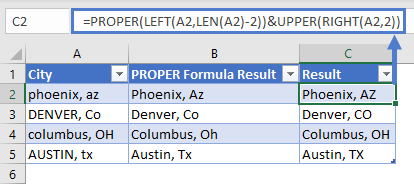The formula uses PROPER, LEFT, and LEN to take the string in column A, trim off the last two characters, and pass the result to the PROPER function. Then, it takes the final two characters of the cell, applies UPPER to them, which converts them to upper case.

You can see the results in column E. Note that this formula will only work where the data is in exactly the same format.

The PROPER Function works exactly the same in Google Sheets as in Excel: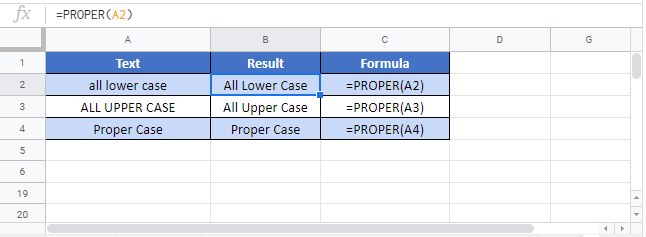## PROPER Examples in VBA

You can also use the PROPER function in VBA. Type:
`application.worksheetfunction.proper(text)`
For the function arguments (text, etc.), you can either enter them directly into the function, or define variables to use instead.